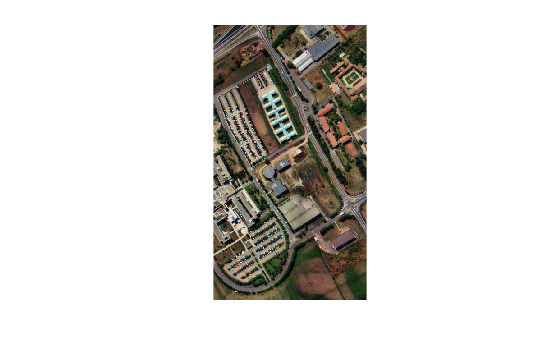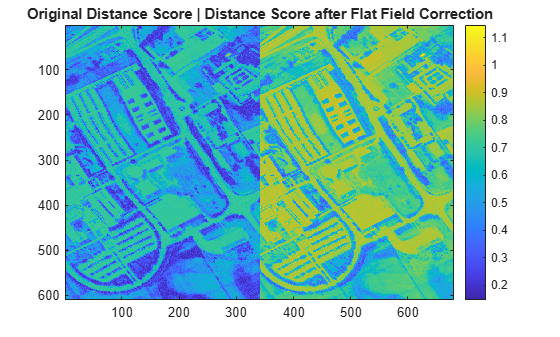# flatField

Apply flat field correction to hyperspectral data cube

Since R2020b

## Syntax

``correctedData = flatField(inputData,roi)``

## Description

example

````correctedData = flatField(inputData,roi)` applies flat field correction to the hyperspectral data, `inputData`, using the flat field mean spectrum calculated in the specified region of interest (ROI) of the hyperspectral data. A valid ROI has these characteristics: Topographically flatSpectrally flat (uniform spectral response)Strong signal source to reduce the impact of random noise NoteThis function requires the Image Processing Toolbox™ Hyperspectral Imaging Library. You can install the Image Processing Toolbox Hyperspectral Imaging Library from Add-On Explorer. For more information about installing add-ons, see Get and Manage Add-Ons.The Image Processing Toolbox Hyperspectral Imaging Library requires desktop MATLAB®, as MATLAB Online™ or MATLAB Mobile™ do not support the library. ```

## Examples

collapse all

Read hyperspectral data into the workspace.

`hcube = hypercube("paviaU");`

Display the contrast-stretched RGB color image of the hyperspectral data.

```coloredImg = colorize(hcube,Method="rgb",ContrastStretching=true); figure imshow(coloredImg)```Specify the ROI from which to calculate the flat field mean spectrum.

`roi = [1 1 10 10];`

Apply the flat field correction to the hyperspectral data.

`hcube_flatfield = flatField(hcube,roi); `

Read the spectral signature of vegetation from the ECOSTRESS spectral library.

```fileroot = matlabshared.supportpkg.getSupportPackageRoot(); filename = fullfile(fileroot,"toolbox","images","supportpackages","hyperspectral","hyperdata", ... "ECOSTRESSSpectraFiles","vegetation.tree.tsuga.canadensis.vswir.tsca-1-47.ucsb.asd.spectrum.txt"); libData = readEcostressSig(filename);```

Compute the distance scores of the spectrum of the original hyperspectral data pixels with respect to the reference spectrum.

`score = spectralMatch(libData,hcube);`

Compute the distance scores of the spectrum of the flat field corrected hyperspectral data pixels with respect to the reference spectrum.

`score_flatfield = spectralMatch(libData,hcube_flatfield);`

Display the distance scores of the original and the flat field corrected hyperspectral data. The pixels with low distance scores are stronger matches to the reference spectrum, and are more likely to belong to the vegetation region. Observe that the distance scores after flat field correction can differentiate between light vegetation cover and dense vegetation cover.

```figure imagesc([score score_flatfield]) colorbar title("Original Distance Score | Distance Score after Flat Field Correction")```## Input Arguments

collapse all

Input hyperspectral data, specified as one of these options:

• `hypercube` object — The `DataCube` property of the `hypercube` object stores the hyperspectral data cube.

• M-by-N-by-C numeric array — M and N are the number of rows and columns of pixels in the hyperspectral data, respectively. C is the number of spectral bands in the hyperspectral data.

The input pixel values can be digital numbers, TOA radiance values, or TOA reflectance values. To convert a hypercube containing digital numbers to a hypercube containing TOA radiance or TOA reflectance data, use the `dn2radiance` or `dn2reflectance` function, respectively.

ROI for the calculation of the flat field mean spectrum, specified as a 4-element vector of positive integers of the form [xmin ymin width height]. The vector defines a rectangular ROI within the hyperspectral data. xmin and ymin are the xy-coordinates of the upper-left corner of the ROI. width and height are the width and height, respectively, of the ROI, in pixels

## Output Arguments

collapse all

Corrected hyperspectral data, returned as a `hypercube` object or M-by-N-by-C numeric array consistent with the input data, `inputData`. If the input data in `inputData` is of data type `double`, then the corrected data is also of data type `double`. Otherwise, the corrected data is of data type `single`.

 Roberts, D. A., Y. Yamaguchi, and R. J. P. Lyon. "Comparison of Various Techniques for Calibration of AIS Data." In Proceedings of the Second Airborne Imaging Spectrometer Data Analysis Workshop, ed. Gregg Vane and Alexander F. H. Goetz, 21 -30. Pasadena: Jet Propulsion Laboratory, 1986.